# Simple Harmonic Motion Formula

Simple Harmonic Motion Formula

Simple Harmonic Motion (SHM) is a special type of periodic motion that follows a certain pattern. The position of an object in simple harmonic motion is described by a sine function that depends on an amplitude of the motion A, an angular frequency, time t, and a starting condition called the phase shift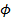. The unit for position and amplitude is meters (m), the unit for angular frequency is radians/s, the unit for time is seconds (s), and the unit for the phase shift is radians.x = position (m)

A = amplitude (m)angular frequency (radians/s)

t = time (s)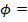phase shift (radians)

Simple Harmonic Motion Formula Questions:

1) A ball on a spring is pulled and released, which sets the ball into simple harmonic motion. The amplitude of the ball's motion is 0.080 m, and the phase shift is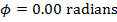. If the angular frequency of the ball's motion is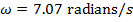, what will be the ball's position at time t = 2.00 s?

Answer: The amplitude of the ball's motion is A = 0.080 m. The position of the ball relative to equilibrium is x, and can be found from the formula: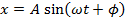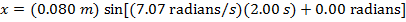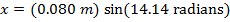x = 0.080 m

At time t = 2.00 s, the ball will be at position x = 0.080 m relative to the equilibrium position.

2) A bee is flying back and forth over a flower, moving in a pattern that can be described by simple harmonic motion. The equilibrium position is the center of the flower. At time t = 0.00 s, the bee is at position x = -0.020 m. The amplitude of the bee's motion is A = 0.020 m, and the angular velocity is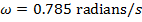. What will be the position of the bee at time t = 4.00 s?

Answer: The first step to solving this problem is to calculate the phase shift. Rather than provide the phase shift value, initial conditions were given. The initial conditions can be used with the simple harmonic motion formula to calculate the phase shift: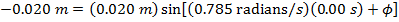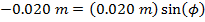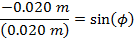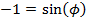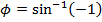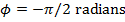The next step to finding the bee's position at time t = 4.00 s is to substitute the known values, including the value of the phase shift, in to the simple harmonic motion formula: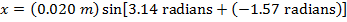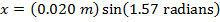x = 0.020 m

The position of the bee at t = 4.00 s is 0.020 m.